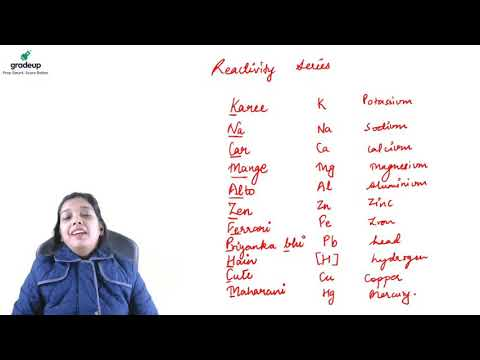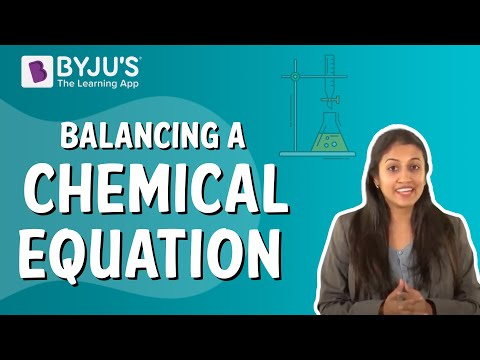Jet Set Go! All about Aeroplanes Jet Set Go! All about Aeroplanes

# Reactivity Series Questions

In Chemistry, we come across some reactions which occur by themselves. The reason for these reactions is the difference in the reactivity/ activity of the involved metals/ metal salts. This series is also found helpful in the extraction of metals.

 Definition: Reactivity Series is the order in which metals are arranged from top to bottom in the decreasing order of their reactivity. This series helps in determining the direction of chemical reaction, the displacement reactions. This series also helps to determine the reaction of metals with acids, water and other chemicals.

## Reactivity Series Chemistry Questions with Solutions

Q1: What happens when you place a Copper strip in a jar containing the MgSO4 solution?

Answer: Since Copper lies below magnesium in the reactivity series, it cannot replace magnesium from its salt solution. Hence, no change is observed when a Cu strip is placed in the MgSO4 solution.

Q2. Which of the following metals will lose an electron first?

1. Li
2. K
3. Cu
4. Zn

Explanation: On ascending from the button to top of the reactivity series, the metal’s ability to lose an electron increases. Since, among the given metals, K lies at the topmost position in the series, K will lose an electron first.

Q3. Why does Iron corrode in the presence of Copper but not in the presence of Zinc?

Answer: On going from bottom to top of the series, the tendency to lose electrons increases and hence, the oxidizing nature of metals increases.

Copper lies below Iron in the reactivity series, hence, Iron is more oxidizable/ reactive metal than copper. Hence, iron corrodes in the presence of copper. While Zinc lies above Iron in the reactivity series and is more reactive than iron. Thus, in the presence of Zinc, iron does not corrode.

Q4. What do metals form upon reaction with water? Give a general chemical reaction for the same.

Answer: Metals on reaction with water from metal hydroxides and hydrogen (gas). The general chemical equation for the reaction is given hereunder.

aM (s) + bH2O → cMx+(OH)x (aq) + dH2 (g)

Q5. Complete the following reaction equations:

1. Metals + water →
2. Metals + O2
3. Metals + acids →

Answer: Metals are highly electropositive elements and have a tendency to oxidize quickly in order to lose electrons. The reaction products of metals with the given reactants are:

1. Metals react with water to form hydrogen and metal hydroxide

Metal + water → Metal hydroxide + H2

1. Metals react with oxygen to form metal oxides.

Metal + O2 → Metal oxide

1. Metals react with acids to form metal salt and H2.

Metal + acid → Salt + H2

Q6. What happens when Mg burns in air? Is this change reversible?

Answer: Mg when burnt in air reacts with oxygen present in the air to form magnesium oxide.

2Mg + O2 → 2MgO

Since the burning of magnesium in air is a chemical change, this change is irreversible.

Q7. What happens when a Cu coin is dropped in water?

Answer: Cu lies beneath H2 in the reactivity series and so, Cu cannot displace H2 from H2O. Hence, no reaction takes place between Cu and water and the copper coin stays as it is.

Cu + H2O → No reaction

Q8. How would you detect the evolution of hydrogen gas from a reaction mixture?

Answer: To detect the liberation of H2 from a reaction mixture, bring a lighted splint is brought close to the mouth of the reaction vessel / test tube, if the splint starts burning with a pop-sound, Hydrogen gas is evolving from the reaction.

Q9. The metals that are less reactive than carbon are extracted by reacting them with carbon. How should the metals that are more reactive than carbon be extracted?

Answer: The metals that are more reactive than carbon can be extracted from their ores by electrolysis. The mineral ore is dissolved into a solution. In this, the carbon electrodes are used and the metal to be extracted gets accumulated at the cathode which carries a negative charge. The then collected purified metal is removed from the solution. By this method, even the trace amounts of metals can be removed from the solution.

Q10. What are Passive metals? Give examples.

Answer: Some metals form a protective oxide layer in order to prevent them from further attack of atmospheric elements such as moisture and air. By this, the metal becomes resistant to corrosion. Thus, the metals other than the noble metals, that have the high resistance to corrosion due to passivity (protective layer formation) are known as Passive metals.

The examples of passive metals are chromium, nickel, tin, aluminium, etc.

Q11. Why is mercury used in thermometers?

Answer: Mercury is the only metal that exists as a liquid at room temperature. Mercury due to having a high coefficient of expansion shows even the slightest of change in the temperature when used in the thermometer.

Q12. Calculate the oxidation state of chromium (Cr) in ammonium dichromate.

Answer: The chemical formula of ammonium dichromate is (NH4)2Cr2O7. As we know, the oxidation state (OS) of neutral compounds is zero. Hence, the sum of the OSs of all the ions present in that compound must be zero.

Therefore, OS((NH4)2) + OS(Cr2O7) = 0

The OS of Cr2O7 can be calculated in terms of Cr and O separately.

Hence, 2 x OS(NH4) + 2 x OS(Cr) + 7 x OS (O) = 0

2 x (+1) + 2 x Cr + 7 x (-2) = 0

2 + 2Cr – 14 = 0

2Cr = 12

Cr = 6

Hence, the OS of Cr in ammonium dichromate is +6.

Q13. During the oxidation of Mg to form MgO, how many electrons are transferred?

Answer: Mg belongs to group 2 of the periodic table and has a +2 OS. The chemical reaction of Mg burning in presence of air is:

2Mg + O2 → 2MgO

To gain a +2 OS, an atom of Mg donates two valence electrons. From the balanced chemical equation of burning of Mg, we can see that 2 moles of Mg are initially involved in the reaction.

Hence, 2 x 2 = 4 electrons are transferred from Mg to oxygen in this reaction.

Q14. Identify the oxidizing agent in the given reaction.

2Au + 4Cl2 → 2AuCl4-2

Answer: In the given reaction, Au goes from 0 OS to +2 OS. Hence, it is oxidized. Cl goes from 0 OS to -1 OS; hence, it is reduced. The substance which itself undergoes reduction in order to oxidize other elements/ compounds is called an oxidizing agent. Hence, in this reaction, Cl is the oxidizing agent

Q15. Which of the following statements about the voltaic cells is not true?

1. Oxidation occurs at the anode
2. Reduction occurs at the cathode
3. Elemental metal is routinely converted into its metal cation at the cathode.
4. None of the above.

Explanation: Metals cations get reduced at the cathode and hence elemental metal is separated.

## Practise Questions on Reactivity Series

Q1. Name two metals that can be extracted by reduction with carbon.

Q2. Consider the following table.

Substance

M.Pt. (oC)

B.Pt. (oC)

Electrical conductivity in solid state

Electrical conductivity in molten/(l) state

A

839

1484

good

good

B

-188

-42

poor

poor

C

776

1497

poor

good

D

-117

78

poor

poor

E

-5

102

poor

good

Which of the above given substances is a metal?

Q3. What is Eocell for the given balanced chemical reaction?

Zn (s) + Pb2+(aq) → Zn2+ (aq) + Pb (s)

Given: For Zn2+ (aq) + 2e → Zn (s); Eo = -0.763

For Pb2+ (aq) + 2e → Pb (s); Eo = -0.126

Q4. Arrange the following halogens in terms of oxidizing property from the lowest oxidizing agent to the weakest oxidizing agent: F2, Cl2, Br2, and I2.

Q5. Write a balanced equation for the reaction of calcium with water.

Click the PDF to check the answers for Practice Questions.

## Reactivity Series## Balancing a Chemical Equation